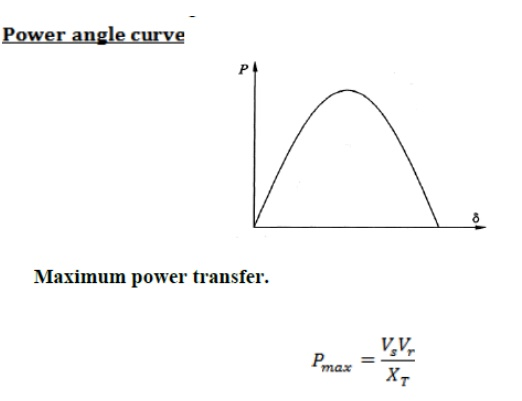Home | | Power System Analysis | Power angle equation and draw the power angle curve

# Power angle equation and draw the power angle curve

Power System Analysis - Stability Analysis - Power angle equation and draw the power angle curve

Power angle equation and draw the power angle curveWhere, P – Real Power in watts

Vs – Sending end voltage;

Vr- Receiving end voltage

XT - Total reactance between sending end receiving end

δ - Rotor angle.Study Material, Lecturing Notes, Assignment, Reference, Wiki description explanation, brief detail
Power System Analysis : Stability Analysis : Power angle equation and draw the power angle curve |